# Determine Compressibility Factor with Present of CO2 and H2S

This example will demonstrate how to determine the compressibility factor (z) for gas with CO2 and H2S. As it is described in the article,Determine Compressibility of Gases , it states that gas with CO2 and H2S must be corrected. This is similar to a normal method for determining z-factor but it is required some correction. Please follow the steps below;

Gas component is shown in Table 1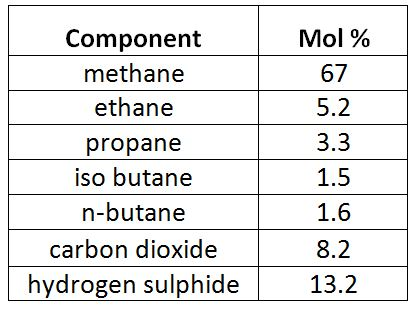Table 1 – Gas Component

Reservoir pressure = 6,000 psia

Reservoir temperature = 190 F

1)   Determine critical pressure and temperature of gas mixtures using Kay’s ruleTable 2 – Critical Pressure and Temperature

Pc‘ = Σyipci = 779.7 psia

Tc’ = ΣyiTci = -22.43 F = -22.43 +460 F = 437.57 RTable 3 – Pc’ and Tr’ by Kay’s Rule

2) Determine ɛ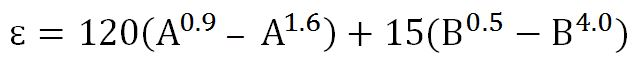Where

A =sum of mole fractions of carbon dioxide (CO2) and hydrogen sulphide (H2S) in the mixture

B = mole fraction of hydrogen sulphide (H2S) in the mixture.

A =0.082 + 0.132 = 0.214

B= 0.1323) Determine corrected pseudo-critical temperature (Tc”) and corrected pseudo-critical pressure (Pc”)

Corrected pseudo-critical temperature (Tc”)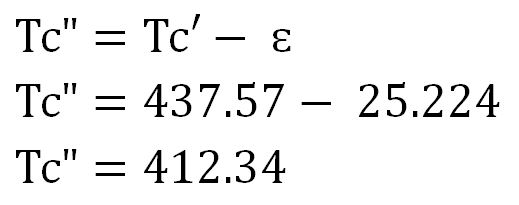Corrected pseudo-critical pressure (Pc”)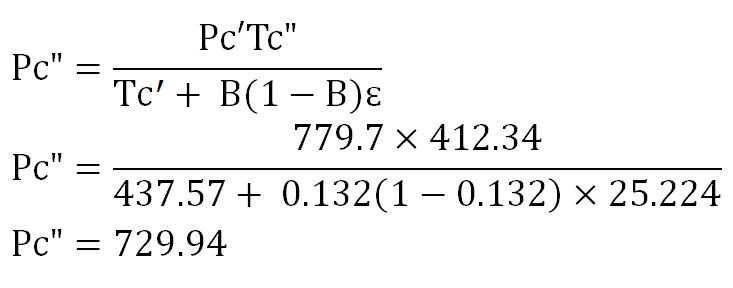4) Calculate pseudo reduced temperature  and pressure

Pr” = P ÷ Pc”

Pr” = 6,000 ÷ 729.94 = 8.22

Tr” = T ÷Tc”

Tr” = (190+460) ÷ (412.34)

Tr” = 1.58

Note: temperature must be in Rankin.

5)   Read the compressibility factor (z) from the chart.

z = 1.022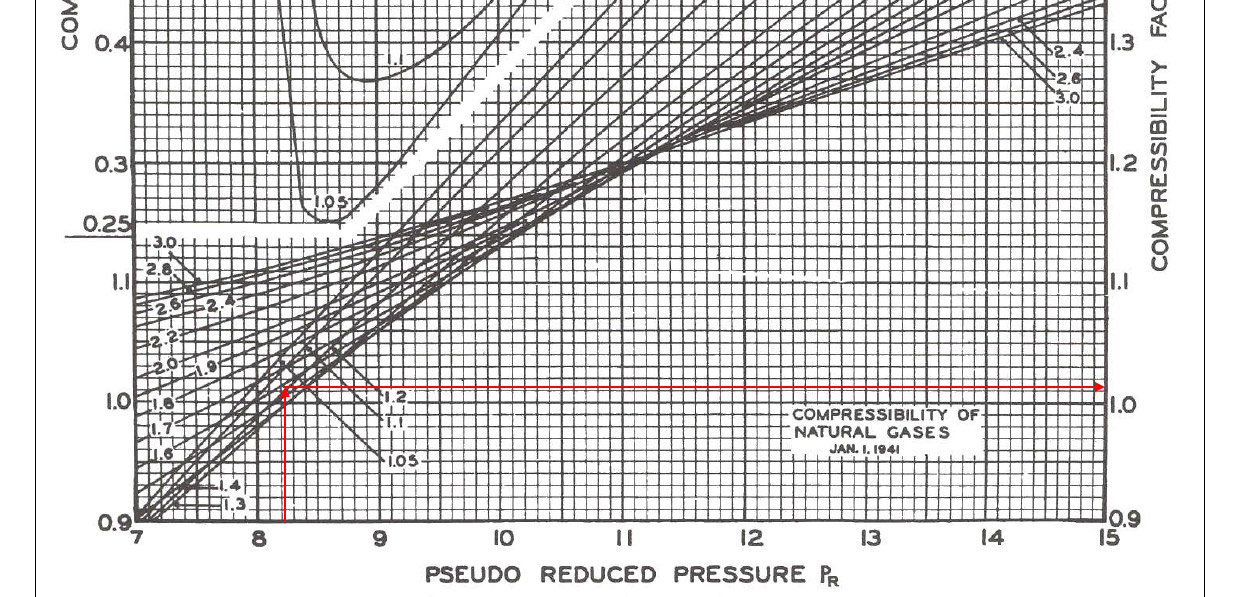Figure 1-z-factor from the Standing and Katz Chart

References

Abhijit Y. Dandekar, 2013. Petroleum Reservoir Rock and Fluid Properties, Second Edition. 2 Edition. CRC Press.

L.P. Dake, 1983. Fundamentals of Reservoir Engineering, Volume 8 (Developments in Petroleum Science). New impression Edition. Elsevier Science.

Tarek Ahmed PhD PE, 2011. Advanced Reservoir Management and Engineering, Second Edition. 2 Edition. Gulf Professional Publishing.

Share the joy
Tagged , , , . Bookmark the permalink.1.Jasmine says: